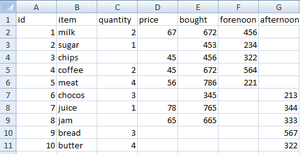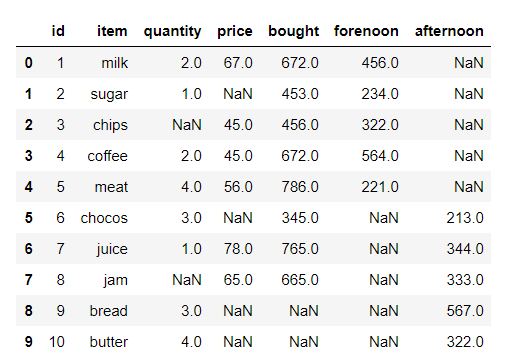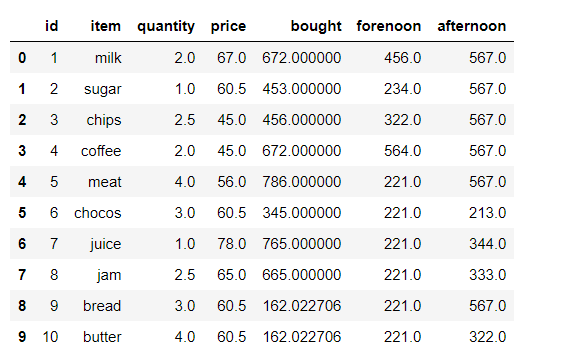Related Articles

# Replacing missing values using Pandas in Python

• Difficulty Level : Basic
• Last Updated : 16 Nov, 2020

Dataset is a collection of attributes and rows. Data set can have missing data that are represented by NA in Python and in this article, we are going to replace missing values in this article

We consider this data set: Dataset

Attention geek! Strengthen your foundations with the Python Programming Foundation Course and learn the basics.

To begin with, your interview preparations Enhance your Data Structures concepts with the Python DS Course. And to begin with your Machine Learning Journey, join the Machine Learning - Basic Level Coursedata set

In our data contains missing values in quantity, price, bought, forenoon and afternoon columns,

So, We can replace missing values in the quantity column with mean, price column with a median, Bought column with standard deviation. Forenoon column with the minimum value in that column. Afternoon column with maximum value in that column.

Approach:

• Import the module
• Fill in the missing values
• Verify data set

Syntax:

Mean: data=data.fillna(data.mean())

Median: data=data.fillna(data.median())

Standard Deviation: data=data.fillna(data.std())

Min: data=data.fillna(data.min())

Max: data=data.fillna(data.max())

Below is the Implementation:

## Python3

 `# importing pandas module``import` `pandas as pd`` ` `# loading data set``data ``=` `pd.read_csv(``'item.csv'``)`` ` `# display the data``print``(data)`

Output:Then after we will proceed with Replacing missing values with mean, median, mode, standard deviation, min & max

## Python3

 `# replacing missing values in quantity``# column with mean of that column``data[``'quantity'``] ``=` `data[``'quantity'``].fillna(data[``'quantity'``].mean())`` ` `# replacing missing values in price column``# with median of that column``data[``'price'``] ``=` `data[``'price'``].fillna(data[``'price'``].median())`` ` `# replacing missing values in bought column with``# standard deviation of that column``data[``'bought'``] ``=` `data[``'bought'``].fillna(data[``'bought'``].std())`` ` `# replacing missing values in forenoon  column with``# minimum number of that column``data[``'forenoon'``] ``=` `data[``'forenoon'``].fillna(data[``'forenoon'``].``min``())`` ` `# replacing missing values in afternoon  column with ``# maximum number of that column``data[``'afternoon'``] ``=` `data[``'afternoon'``].fillna(data[``'afternoon'``].``max``())`` ` `print``(Data)`

Output:My Personal Notes arrow_drop_up